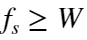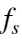## Verification of Sampling Theorem

Version 1.0.0 (1.46 KB) by
Aim: To verify Sampling theorem for a signal of given frequency -To look at conditions of oversampling and undersampling

Updated 30 Nov 2021

Theory:
• Sampling is a process of converting a continuous time signal (analog signal) x(t) into a discrete time signal x[n], which is represented as a sequence of numbers. (A/D converter)
The sampling theorem specifies the minimum-sampling rate at which a continuous-time signal needs to be uniformly sampled so that the original signal can be completely recovered or reconstructed by these samples alone. This is usually referred to as Shannon's sampling theorem in the literature.or, in term of the sampling periodThe minimum sampling rate allowed by the sampling theorem (= 2W) is called the Nyquist rate.
Algorithm:
1. Input the desired frequency fd (for which sampling theorem is to be verified).
2. Generate an analog signal xt of frequency fd for comparison.
3. Generate oversampled, nyquist & under sampled discrete time signals.
4. Plot the waveforms and hence prove sampling theorem.
MATLAB Implementation:
Step 1: MATLAB can generate only discrete time signals. For an approximate analog signal xt, choose the spacing between the samples to be very small (≈0), say 50µs = 0.00005. Next choose the time duration, say xt exists for 0.05seconds.(tfinal in program) (for low frequency say <1000 Hz choose 0.05 secs, for higher choose 0.01 secs or lesser as appropriate). Now begin with the vector that represents the time base= t = 0:0.00005:0.05; The colon (:) operator in MATLAB creates a vector, in the above case a time vector running from 0 to 0.05 in steps of 0.00005. The semicolon (;) tells MATLAB not to display the result. Given t, the analog signal xt of frequency fd is generated as (sin(ωt)=sin(2πft))- xt=sin(2*pi*fd*t); pi is recognized as 3.14 by MATLAB
Step 2: To illustrate oversampling condition, choose sampling frequency fs0=2.2*fd. For this sampling rate T0=1/fs0, generate the time vector as n1 = 0:T0:0.05; & over sampled discrete time signal x1=sin(2*pi*fd*n1); [Alternately let n1 be a fixed number of samples, say n=0:10; & x1=sin(2*pi*n*fd/fs0);] The discrete signal is converted back to analog signal (reconstructed) using the MATLAB plot function (FOH). In one plot both the analog signal (approximate one in blue color) and the reconstructed signal (in red) are plotted for comparison
Step 3: Repeat step 2 for different sampling frequencies, i.e., fs=1.3*fd & fs=2*fd for undersampling and Nyquist sampling conditions.

### Cite As

Nishita Anand (2023). Verification of Sampling Theorem (https://www.mathworks.com/matlabcentral/fileexchange/102744-verification-of-sampling-theorem), MATLAB Central File Exchange. Retrieved .

##### MATLAB Release Compatibility
Created with R2021b
Compatible with any release
##### Platform Compatibility
Windows macOS Linux